Next: Eigenstates of Angular Momentum Up: Orbital Angular Momentum Previous: Angular Momentum Operators

# Representation of Angular Momentum

Now, we saw earlier, in Sect. 7.2, that the operators,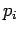, which represent the Cartesian components of linear momentum in quantum mechanics, can be represented as the spatial differential operators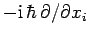. Let us now investigate whether angular momentum operators can similarly be represented as spatial differential operators.

It is most convenient to perform our investigation using conventional spherical polar coordinates: i.e.,,, and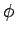. These are defined with respect to our usual Cartesian coordinates as follows: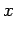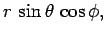(545)(546)(547)

It follows, after some tedious analysis, that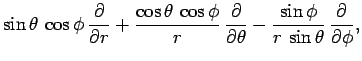(548)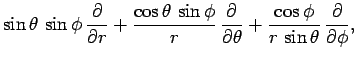(549)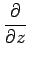(550)

Making use of the definitions (527)-(529), (534), and (538), the fundamental representation (478)-(480) of theoperators as spatial differential operators, the Eqs. (545)-(550), and a great deal of tedious algebra, we finally obtain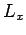(551)(552)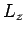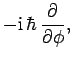(553)

as well as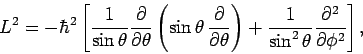(554)

and(555)

We, thus, conclude that all of our angular momentum operators can be represented as differential operators involving the angular spherical coordinates,and, but not involving the radial coordinate,.Next: Eigenstates of Angular Momentum Up: Orbital Angular Momentum Previous: Angular Momentum Operators
Richard Fitzpatrick 2010-07-20